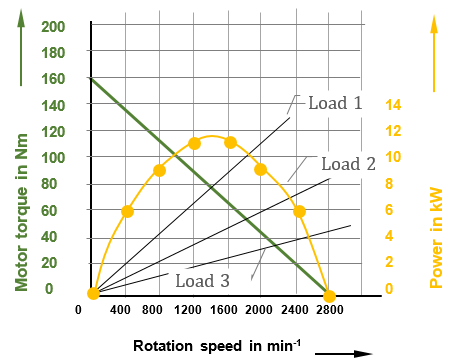# Work order pneumatic motor – Solution

Proposed solution:

The following measuring table serves to determine the performance curve:Note: The mechanical power can be calculated by:  Pmech = T * rpm / 9550

From the performance curve (yellow curve) of the motor, it can be seen that at 1400 rpm it has its optimum performance. Thus, the operating point (Δ intersection point of the motor torque and load characteristic curve) is optimal when the load 1 is selected.fig.: pneumatic motor curve